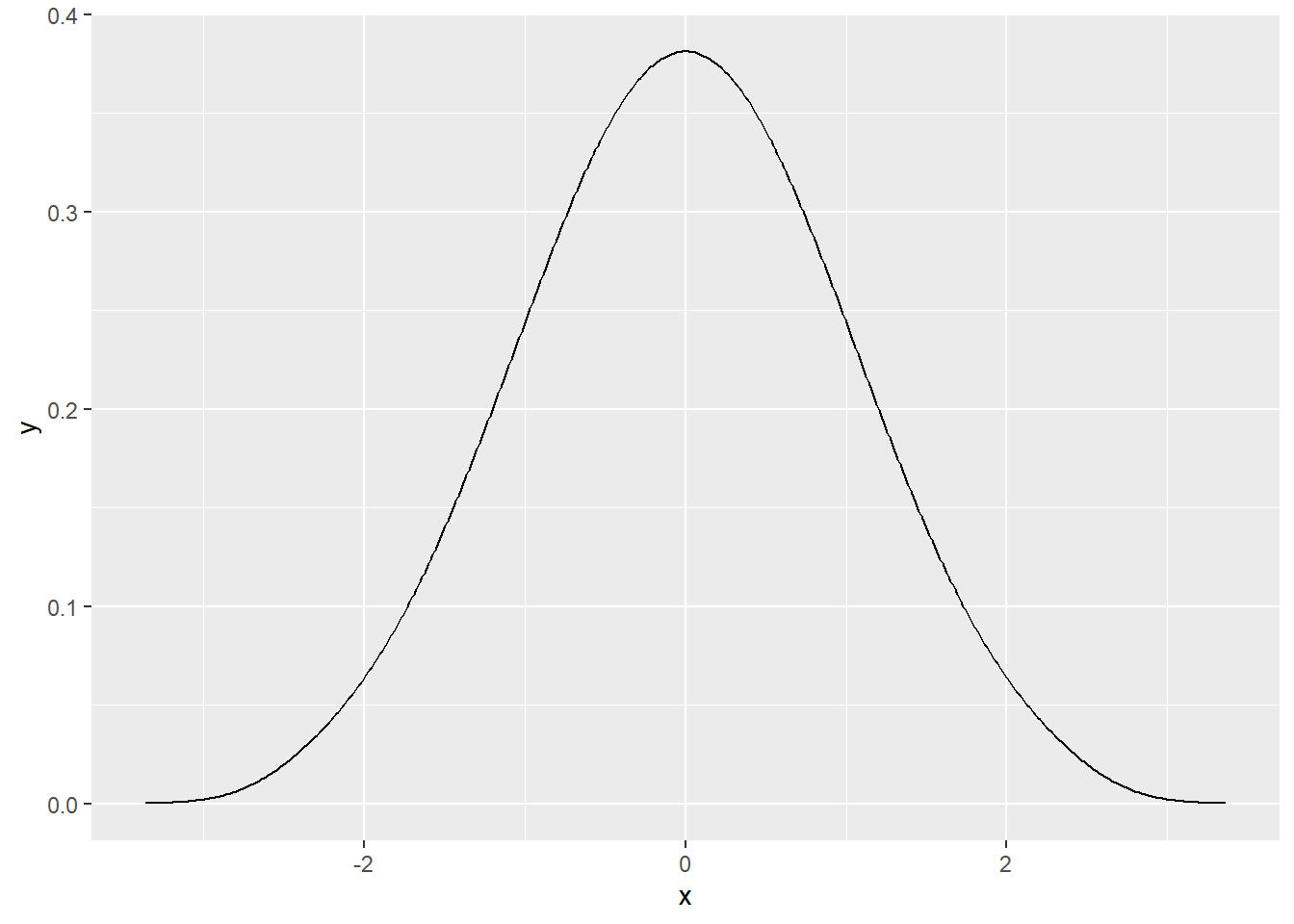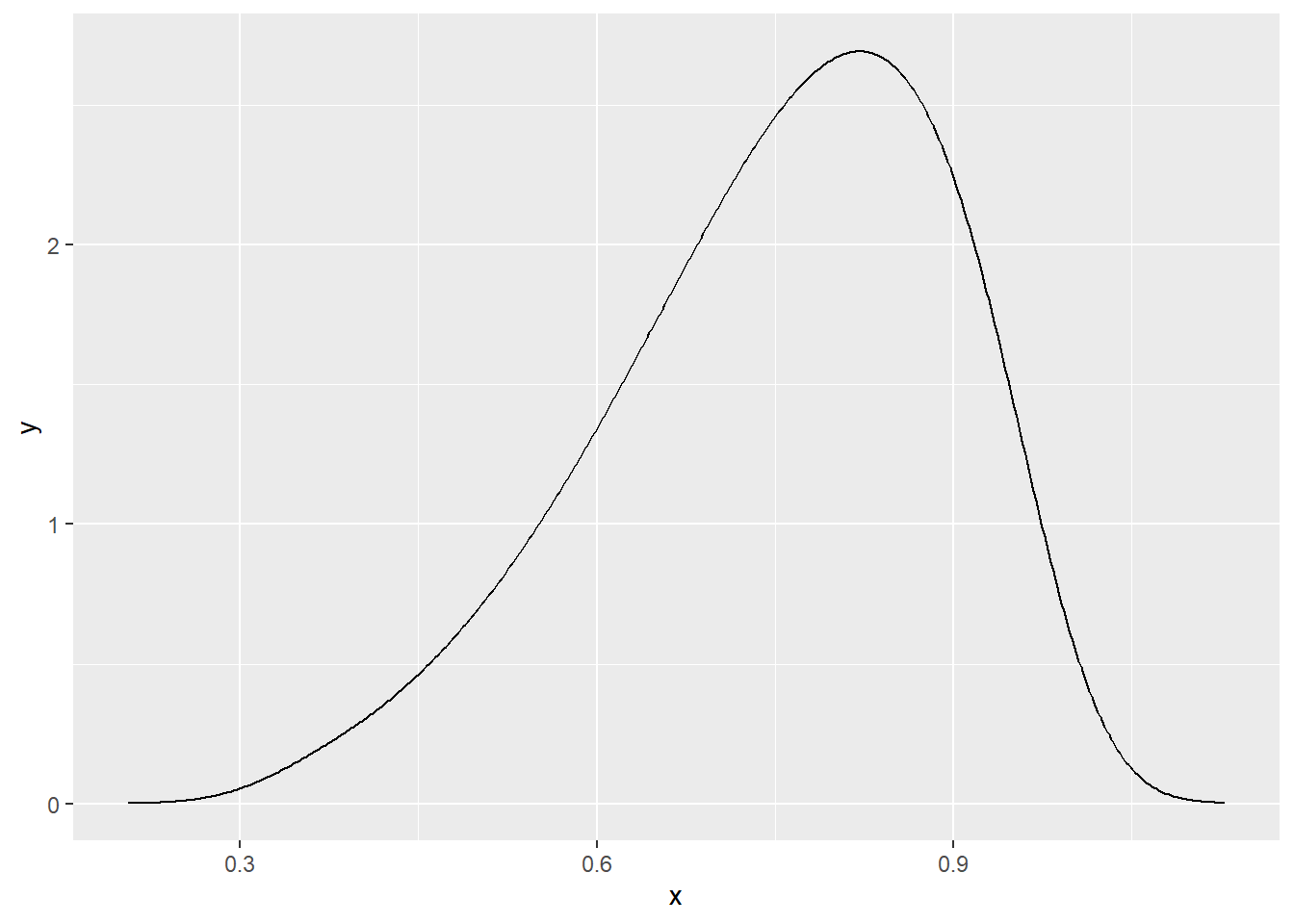# A perfectly normally distributed sample: another post?

Recently, we published a post presenting a small convenience function, from the bayestestR package. This function, named `rnorm_perfect`, generated an empirical distribution (i.e., a vector of values) as close as possible to a desired distribution, in this case the normal distribution.

It worked like this:

``````# Generate a perfect sample
x <- rnorm_perfect(n = 100, mean = 0, sd = 1)

# Visualise it
library(tidyverse)

x %>%
density() %>%  # Compute density function
as.data.frame() %>%
ggplot(aes(x=x, y=y)) +
geom_line()``````This function’s name was initially chosen because of its proximity (argument-wise) with its random counterpart `rnorm` (it has the same arguments, the same order and the same output type). However, we quickly realised this this name was not perfect (no pun intended), as indeed the obtained distribution was not random (and the `r` in `rnorm` stands for random).

Thus, after discussion, we decided to change it to `distribution_normal`. We also used that opportunity to add other types of “perfect” distributions:

``````# Generate a perfect sample
x <- distribution_beta(n = 100, 6, 2)

x %>%
density() %>%
as.data.frame() %>%
ggplot(aes(x=x, y=y)) +
geom_line()``````Interestingly, a few days before this update, we received a small email asking:

Hi, I saw your blog post and wonder how you define a perfectly normal distribution.

We responded:

that’s actually a good question. I would say an empirical sample having characteristics as close as possible to a canonic Gaussian distribution.

After an (ironic?) “Thanks, most helpful!”, this blog post got published, which emphasize on the irrelevance of the `r` prefix in the function name. Thanks to some of the good points raised in this post, we’ve felt the need to update users on this function.

Thus, please note that the `rnorm_perfect` name will be deprecated in the next version, and ultimately removed to avoid further confusion :)

## Any other suggestions?

Don’t forget, the easystats project (that includes `bayestestR`) is very open to contributions! Remember that you can always make suggestions and contribute to the package, to actually help improving it, with the spirit of collaboration, and in the tradition of open science ;)

## Get involved

Feel free to let us know how we could further improve this package! Also, note that easystats, the project supporting `bayestestR` is in active development. Thus, do not hesitate to contact us if you want to get involved :)

• Check out our other blog posts here!